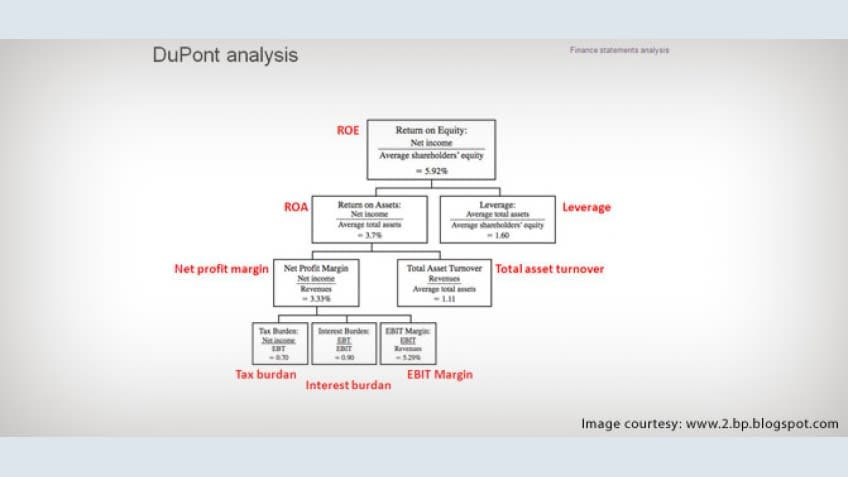DuPont analysis is an important topic which is covered in CFA Level 1 exam under financial reporting and analysis. In this article, I will discuss the concept of DuPont Analysis. We will also discuss how we can perform DuPont Analysis using MS Excel. We will try to build a simple model for performing calculations for DuPont Analysis.

DuPont analysis is an approach which is used to analyze return on equity (ROE). It uses simple algebra to breakdown ROE into a function of different ratios so that the impact of leverage, profit margin and turnover on shareholders return can be studied.

There are two versions of DuPont Analysis:-

1. Original three part approach
2. Extended five part approach

Let us define ROE:-

Multiplying and dividing by Revenue on the right hand side of the above function, we get

We can further expand this function by multiplying and dividing by Assets on the right hand side of the above function

In the above function, the first term is profit margin, second term is asset turnover, and the third term is leverage ratio which will increase if debt is used to finance the assets. The above function can be rewritten as:-

This is the original three part DuPont equation. If the ROE is low as compared to the previous year, then at least one of the following conclusions is definitely true:-

• Company has poor profit margin.
• Company has poor asset turnover.
• Firm has too little leverage.

In the extended five part approach, the net profit margin can be broken up as shown in the equation below:-

We can give names to ratios in the above equation. The above equation can be written as follows:-

This is the extended five part DuPont Equation.

Now I will discuss an example problem on DuPont Analysis and try to make small model of this problem on MS Excel.
Example - Balance sheet and Income Statement Items of company A are given below (All figures are in millions)

 Year 2010 2011 2012 Net Income 20 22 21 Sales 302 330 390 Equity 125 130 132 Assets 215 250 300

We can calculate ROE from the above table. We can also calculate the other ratios present in the original three part DuPont equation. We will use MS excel to perform all these calculations.

Step 1 - Enter all the data given in the table in MS Excel spreadsheet.

Step 2 - Now create another table in MS Excel spreadsheet as shown in the picture below.

Step 3 - Now we have to calculate ROE and other Ratios as mentioned in the table. It can seen that Net Income for year 2010 is present in Cell C3 and Equity is present in Cell C5. Now we can calculate the ROE for year 2010 by using these two figures. Go to Cell C11 in excel spreadsheet and insert equal to (=) sign. Then select cell C3 and C5 separated by division sign (/). Then press enter to get the ROE value. Refer the picture below for more details:-

Step 4 - Similarly, ROE for year 2011 and 2012 can be calculated. Also all the other ratios in the table can be calculated by selecting the appropriate cells.

Analysis of the ratios:-

• ROE has slightly gone up and then decreased slightly.
• Net Profit Margin has declined slightly.
• Asset turnover has decreased in two years.
• Leverage ratio has gone up in two years.Eshna